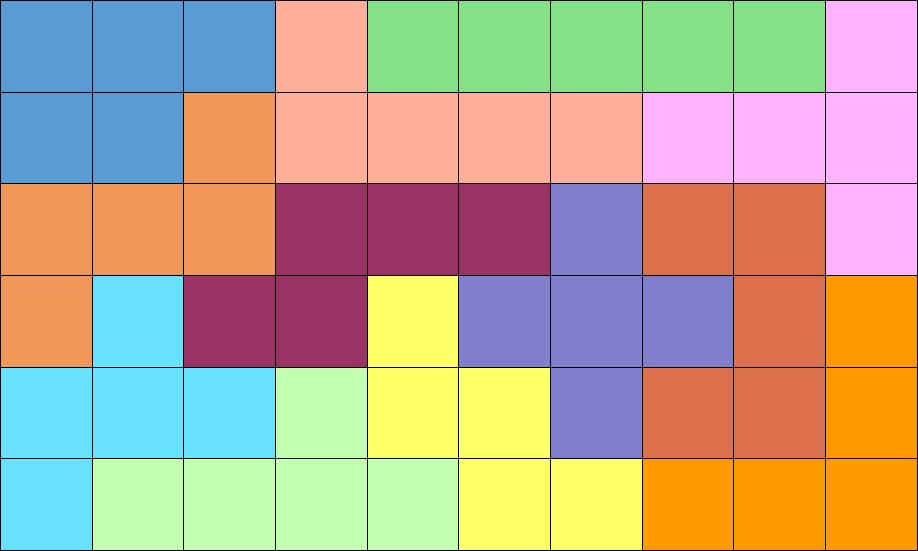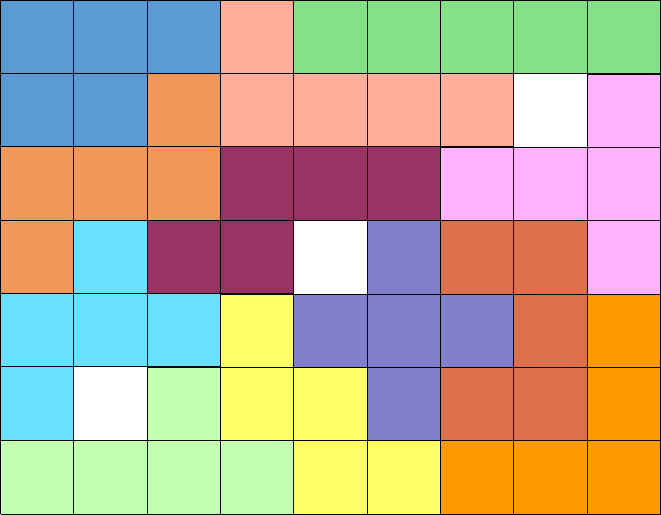Top of page

# Mathematical Games of Martin Gardner Part 4

April is Mathematics and Statistics Awareness Month (MSAM) and we are continuing with our weekly nod to recreational mathematics inspired by “Mathematical Games,” a monthly column that appeared in Scientific American between 1956 and 1981. This column was authored by Martin Gardner, a tireless advocate for mathematics education and mathematical games. This week’s puzzle features the cryptarithm.

A cryptarithm is a mathematical puzzle where numbers 0-9 have been replaced by letters with the exercise being solved through simple arithmetic. When the letters form words it can also be known as an alphametic. A wonderful example that is cited quite frequently is Henry E. Dudeney’s “Perplexities” column that appeared in the July 1924 issue of The Strand Magazine. Dudeney provides 4 puzzles, 1 each for addition, subtraction, multiplication, and division. Dudeney’s example of a cryptarithm involving addition can be seen here:

SEND
+MORE
MONEY

Solution to Dudeney’s cryptarithm SEND + MORE = MONEY:

9567 + 1085 = 10652

In this example, we know the sum of the S from SEND and the M from MORE must equal 10 or more because of the additional column in the answer. To get the carryover digit in the 5th column, the sum of S and M must equal at least 10. The highest the carryover could be is 1 so we know that M = 1 which means S = 9 and O translates to 0. This also means that there is no carryover from the 3rd column.

We know that each letter represents a unique number so E cannot equal N, which means there is a carryover of 1 from the 2nd column. This column can now be written as E + 1 + 0 = N. And because of that carryover we have 2 possibilities for the 2nd column: N + R = 10 + E (no carryover from the 1st column) or N + R + 1 = 10 + E (carryover of 1 from the 1st column). Substituting the N with E + 1 will change these to: E + 1 + R = 10 + E and E + 1 + R + 1 = 10 + E. In both, the Es will cancel out and after solving for R we are left with either R = 9 or R = 8. Since S = 9, R must equal 8 and we now know that there is a carryover from the 1st column.

So far we have M = 1; S = 9; O = 0; and R = 8. Focusing on the 1st column, we know that D + E must be equal to or greater than 12 to account for the carryover and because Y cannot be 0 (O) or 1 (M). D and E also cannot be 8 (R) or 9 (S) as those numbers have already been paired with letters so this leaves 5 or 6 + 7 as possibilities.

If E = 7, then N would be 7 + 1, or 8. We just found that R = 8 so we know E cannot equal 7 regardless of whether it’s paired with 5 or 6. If D = 7 and E = 6, then N would be 6 + 1, or 7. And because D cannot equal E, we now have N = 5 + 1, or 6.

This is a lot, but we’re almost there! With D = 7 and E = 5 (added together to be 12) we know that Y = 2, with the 1 being carried over to the 2nd column. So altogether, we have:

M = 1; S = 9; O = 0; E = 5; N = 6; R = 8; D = 7; and Y = 2.

This week’s puzzle also uses addition:

GOOD
+ TIME
MATHS

There are multiple solutions to this puzzle so let us know in the comments how you found yours. Hint: O = 3. And if this has inspired you to create your own, share those with us and we’ll try them out!

Last week’s puzzle dealt with polyominos. The object of the exercise was to split the 6 x 10 rectangle into 2 pieces, without splitting any of the polyominos, and to reform them into a 7 x 9 rectangle with 3 holes. The original 6 x 10 rectangle is shown here with the solution appearing below:12 pentominoes arranged in a 6 x 10 rectangle.The solution to last week’s puzzle shows how the original 6 x 10 rectangle can be divided into 2 pieces and reformed into a 7 x 9 rectangle.

To get this rectangle, the original is split diagonally from top-right to bottom-left. The two pieces are then moved down 1 space and to the left 1 space so that 3 holes appear as shown.

As with previous weeks, this week’s solution will appear in next week’s post. If you take part in any mathematical activities during the month of April, be sure to use #MathStatMonth for posts on social media. Good luck and share your experience with us in the comments and if you’d like, share some of your favorite cryptarithms for us to solve!

Do you want more stories like this? Then subscribe to Inside Adams — it’s free!